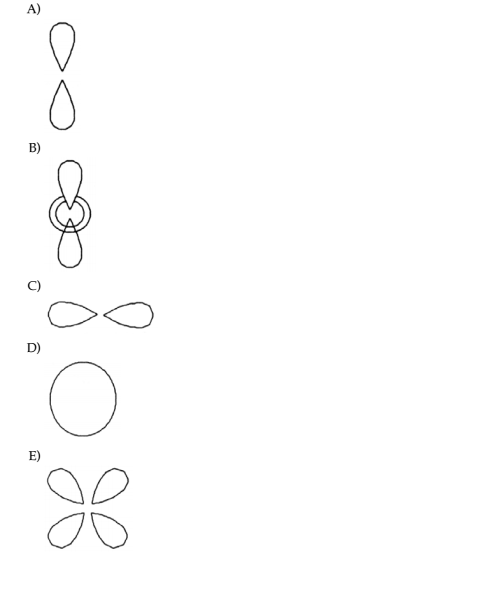# Problem: Which sketch represents an orbital with the quantum numbers n = 3, l = 0, and ml = 0?

84% (33 ratings)
###### Problem Details

Which sketch represents an orbital with the quantum numbers n = 3, l = 0, and ml = 0?What scientific concept do you need to know in order to solve this problem?

Our tutors have indicated that to solve this problem you will need to apply the Orbital Shapes concept. You can view video lessons to learn Orbital Shapes. Or if you need more Orbital Shapes practice, you can also practice Orbital Shapes practice problems.

What is the difficulty of this problem?

Our tutors rated the difficulty ofWhich sketch represents an orbital with the quantum numbers ...as low difficulty.

How long does this problem take to solve?

Our expert Chemistry tutor, Jules took 1 minute and 7 seconds to solve this problem. You can follow their steps in the video explanation above.

What professor is this problem relevant for?

Based on our data, we think this problem is relevant for Professor Tang's class at USF.